Introduction
Recently, I was implementing skin shading in my engine. The pre-integrated skin shading technique is chosen because it has a low performance cost and does not require another extra pass. The idea is to pre-bake the scattering effect over a ring into a texture for different curvature to look up during run-time. More information can be found in the GPU Pro 2, the SIGGRAPH slides, and also in the presentation of the game "The Oder:1886". Here is the result implemented in my engine (all screen shots are rendered with filmic tone mapping):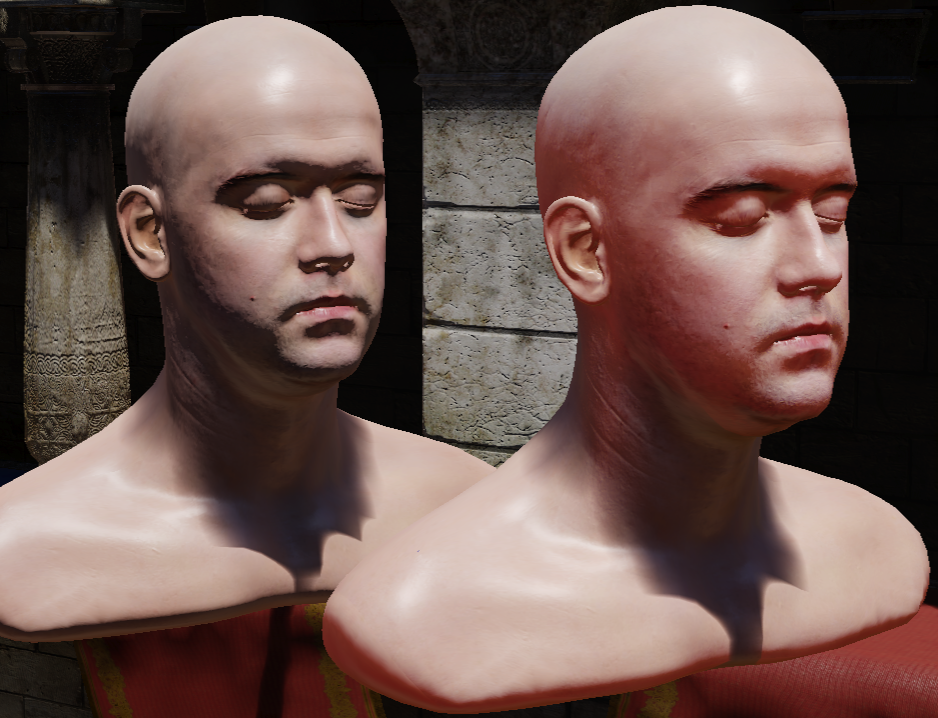The head on the left is lit with Oren-Nayar shading The head on the right is lit with pre-integrated skin

Curve Approximation for Direct Lighting
In my engine, iOS is one of my target platform, which only have 8 texture unit to use for the OpenGL ES 2.0 API. This is not enough for the pre-integrated skin look up texture because my engine have already used some slots for light map, shadow map, IBL... So I need to find an approximation to the look up texture.

Unfortunately I don't have a Mathematica license at home. So I think may be I can fit the curve manually by inspecting the shape of the curve. So, I started by plotting the graph of the equation:
Here is the shape of the red channel diffusion curve, plotting with N.L(normalized to [0, 1])and r (from 2 to 16):
My idea for approximating the curve is by finding some simple curves first and then interpolate between them like this:
For the light blue line in the above figure, a single straight line can get a close enough approximation:
curve1= saturate(1.95 * NdotL -0.96)
To approximate the dark blue line, I divide it into 2 parts: linear part and quadratic part:
curve0_linear= saturate(1.75* NdotL -0.76)
blending both linear and quadratic curve will get a curve that is similar to the original function:
Now we have 2 curves that is similar to our original function at both end. By mixing them together, we can get something similar to the original function like this:
curve= lerp(curve0, curve1, 1 - (1 - curvature)^4)
By repeating the above steps for the blue and green channels, we can shade the pre-integrated skin without the look up texture. Here is the result:

This is how it looks like when applying to a human head model:

For your reference, here is the approximated function I used for the RGB channels:
NdotL = mad(NdotL, 0.5, 0.5); // map to 0 to 1 range
float curva = (1.0/mad(curvature, 0.5 - 0.0625, 0.0625) - 2.0) / (16.0 - 2.0); // curvature is within [0, 1] remap to normalized r from 2 to 16
float oneMinusCurva = 1.0 - curva;
float3 curve0;
{
float3 rangeMin = float3(0.0, 0.3, 0.3);
float3 rangeMax = float3(1.0, 0.7, 0.7);
float3 offset = float3(0.0, 0.06, 0.06);
float3 t = saturate( mad(NdotL, 1.0 / (rangeMax - rangeMin), (offset + rangeMin) / (rangeMin - rangeMax)  ) );
float3 lowerLine = (t * t) * float3(0.65, 0.5, 0.9);
lowerLine.r += 0.045;
lowerLine.b *= t.b;
float3 m = float3(1.75, 2.0, 1.97);
float3 upperLine = mad(NdotL, m, float3(0.99, 0.99, 0.99) -m );
upperLine = saturate(upperLine);
float3 lerpMin = float3(0.0, 0.35, 0.35);
float3 lerpMax = float3(1.0, 0.7 , 0.6 );
float3 lerpT = saturate( mad(NdotL, 1.0/(lerpMax-lerpMin), lerpMin/ (lerpMin - lerpMax) ));
curve0 = lerp(lowerLine, upperLine, lerpT * lerpT);
}
float3 curve1;
{
float3 m = float3(1.95, 2.0, 2.0);
float3 upperLine = mad( NdotL, m, float3(0.99, 0.99, 1.0) - m);
curve1 = saturate(upperLine);
}
float oneMinusCurva2 = oneMinusCurva * oneMinusCurva;
float3 brdf = lerp(curve0, curve1, mad(oneMinusCurva2, -1.0 * oneMinusCurva2, 1.0) );

Curve Approximation for Indirect Lighting
In my engine, the indirect lighting is stored in spherical harmonics up to order 2. So the pre-integrated skin BRDF need to be projected into spherical harmonics coefficients and can be store in look up texture just like the presentation of "The Oder:1886" described. One thing to note about the integral range in equation (19) from the paper should be up to π instead of π/2 because to project the coefficients, we need to integrate over the whole sphere domain and the value of D(θ, r) in the lower hemi-sphere may be non zero for small r due to sub-surface scattering, which does not like the clamped cos(θ). So I compute the spherical harmonics coefficient using this:
To make the indirect lighting work on my target platform, an approximate function to this indirect lighting look up texture also need to be found. By using similar methods described above and with some trail and error. Here is my result:

And applying it to the human head model, but this time the approximation is not close enough and lose a bit of red color:

And some code for your reference, where the ZH is the zonal harmonics coefficient:
float curva = (1.0/mad(curvature, 0.5 - 0.0625, 0.0625) - 2.0) / (16.0 - 2.0); // curvature is within [0, 1] remap to r distance 2 to 16
float oneMinusCurva = 1.0 - curva;
// ZH0
{
float2 remappedCurva = 1.0 - saturate(curva * float2(3.0, 2.7) );
remappedCurva *= remappedCurva;
remappedCurva *= remappedCurva;
float3 multiplier = float3(1.0/mad(curva, 3.2, 0.4), remappedCurva.x, remappedCurva.y);
zh0 = mad(multiplier, float3( 0.061659, 0.00991683, 0.003783), float3(0.868938, 0.885506, 0.885400));
}
// ZH1
{
float remappedCurva = 1.0 - saturate(curva * 2.7);

float3 lowerLine = mad(float3(0.197573092, 0.0117447875, 0.0040980375), (1.0f - remappedCurva * remappedCurva * remappedCurva), float3(0.7672169, 1.009236, 1.017741));
float3 upperLine = float3(1.018366, 1.022107, 1.022232);
zh1 = lerp(upperLine, lowerLine, oneMinusCurva * oneMinusCurva);
}
Result
Putting both the direct and indirect lighting calculation together, with a simple GGX specular, lit by 1 directional light, SH projected indirect light and pre-filtered IBL.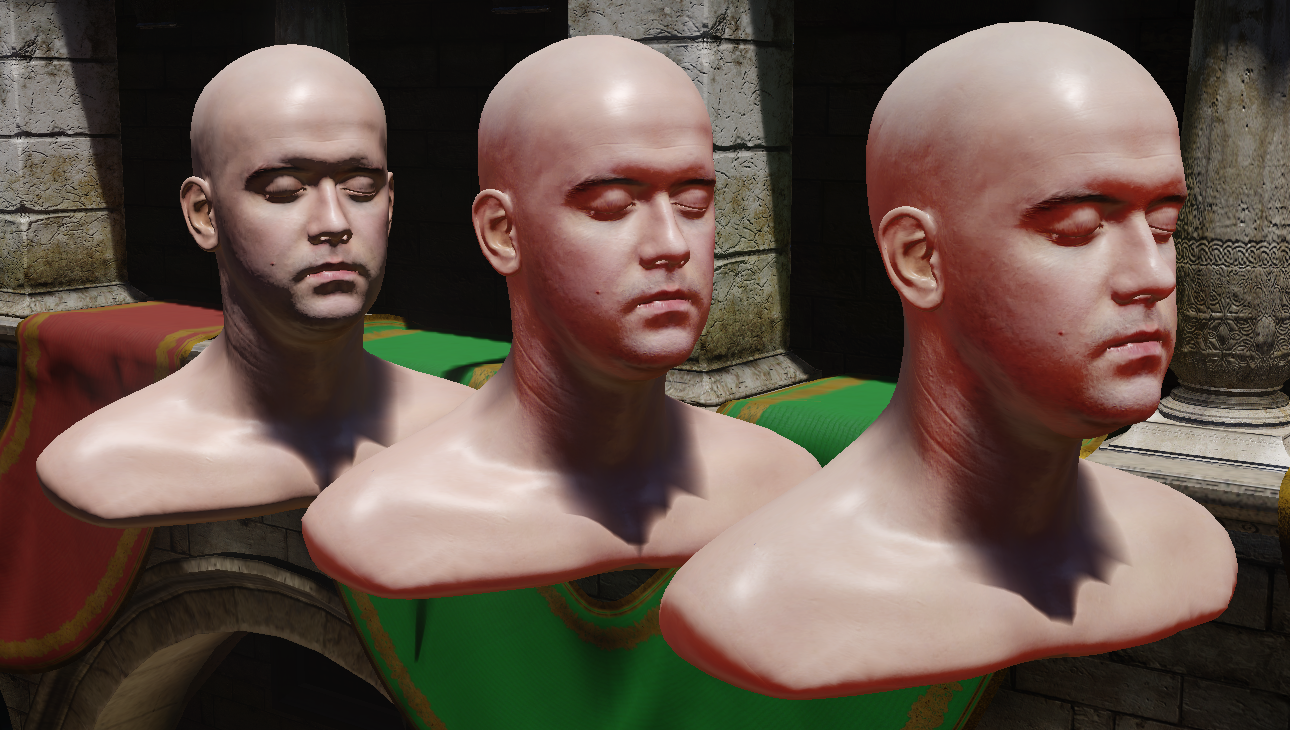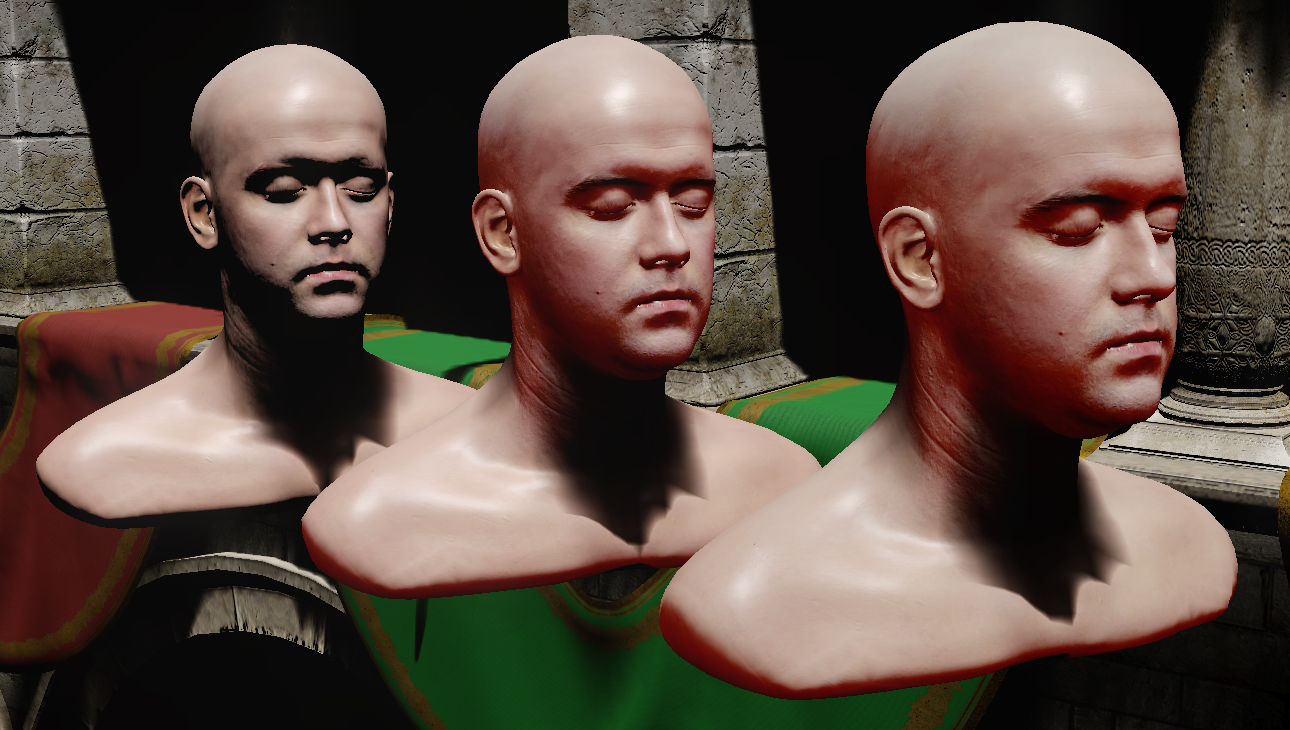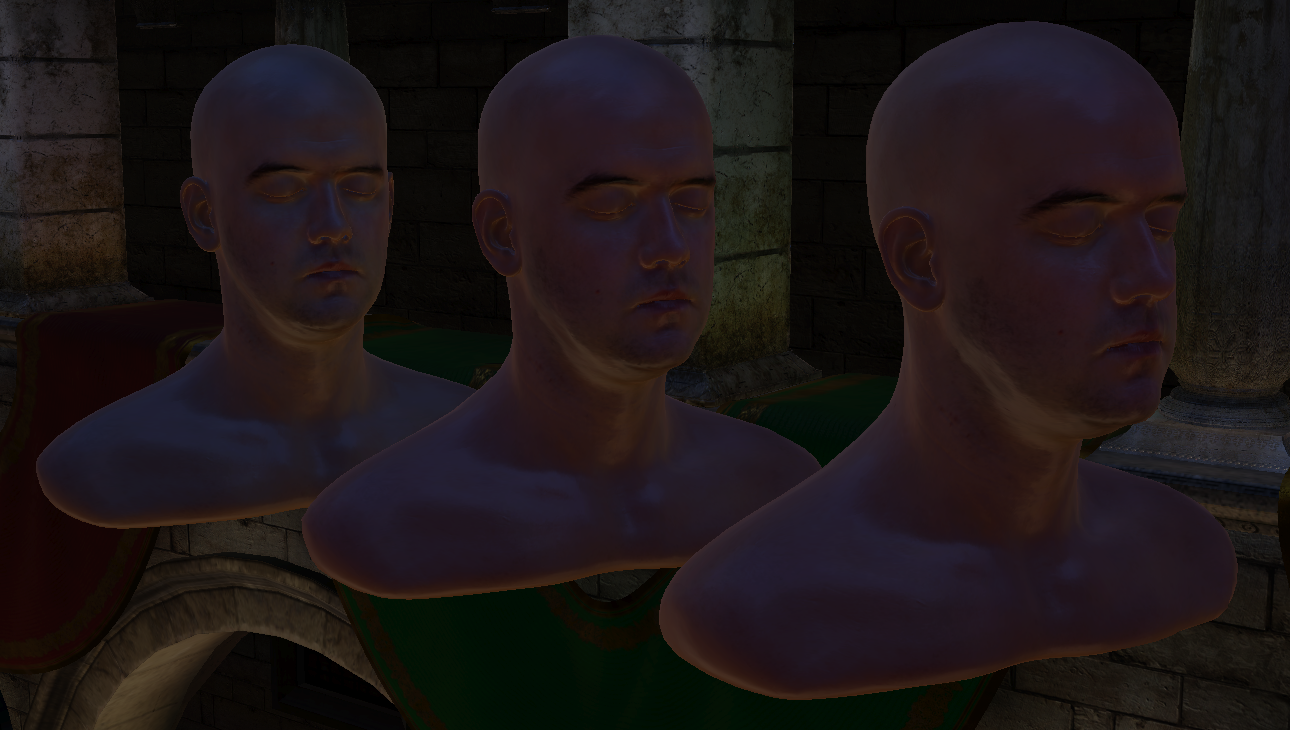Heads from left to right: shaded with lambert shader, approximated function, look up texture Images from left to right: full shading, direct lighting only, indirect lighting only Upper row: shaded with albedo texture applied Lower row: showing only lighting result

With another lighting environment: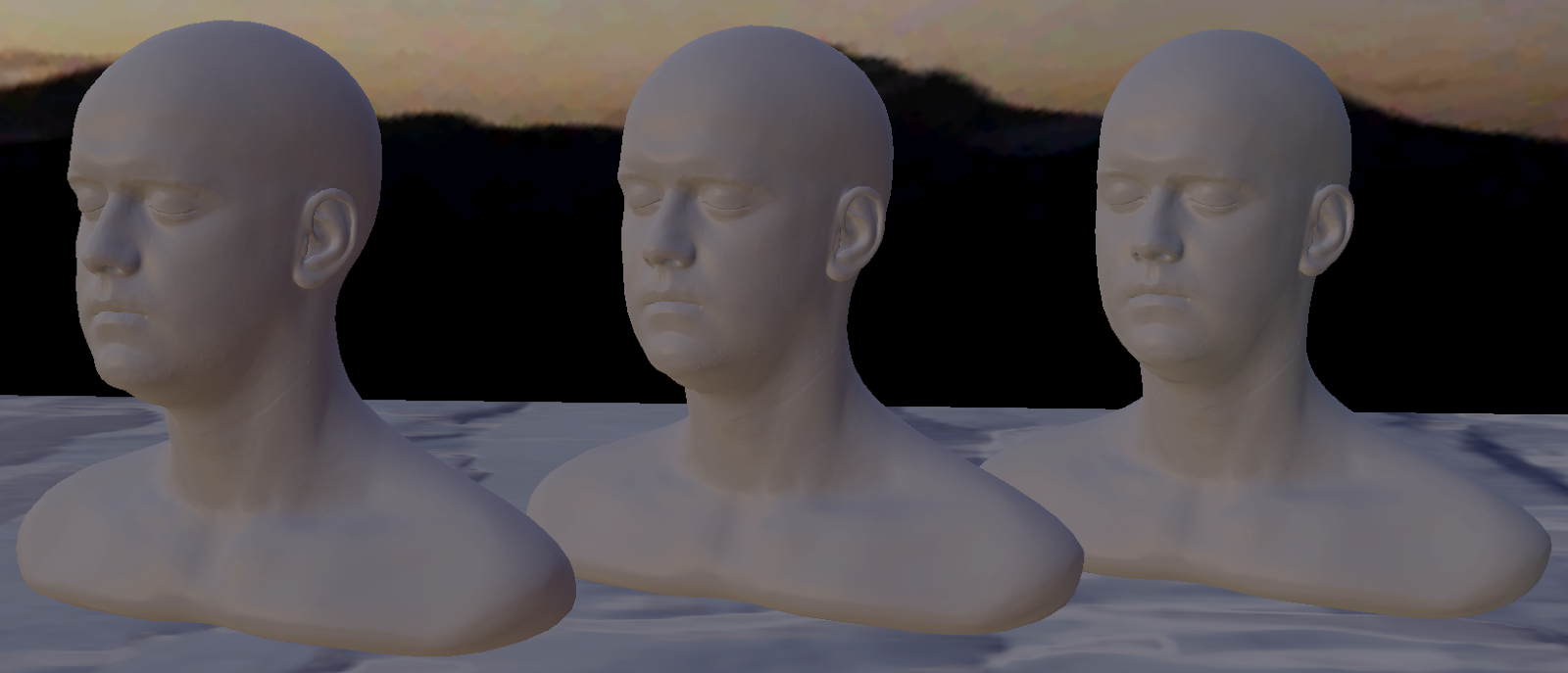Heads from left to right: shaded with look up texture, approximated function, lambert shader Images from left to right: full shading, direct lighting only, indirect lighting only Upper row: shaded with albedo texture applied Lower row: showing only lighting result

I have uploaded the curvature map for the human head here, look up texture for the direct lighting here and indirect lighting look up texture here. The textures need to be loaded without sRGB conversion. For the indirect lighting texture, I have scale the value so that it fits into an 8-bit texture within 0 to 1 range. So a sample use of the look up textures looks like:
float3 brdf= directBRDF.Sample(samplerLinearClamp, float2(mad(NdotL, 0.5, 0.5), oneOverR)).rgb;
float3 zh0= indirectBRDF_ZH.Sample(samplerLinearClamp, float2(oneOverR, 0.25)).rgb;
float3 zh1= indirectBRDF_ZH.Sample(samplerLinearClamp, float2(oneOverR, 0.75)).rgb;
float remapMin= 0.75;
float remapMax= 1.05;
zh0= zh0 * (remapMax - remapMin) + remapMin;
zh1= zh1 * (remapMax - remapMin) + remapMin;
Conclusion
In this post, I describe a way to find an approximated function for the pre-integrated skin diffusion profile, which gives a similar result for the direct lighting function while losing a bit of red color for the indirect lighting. The down side of fitting the curve manually is when the function is changed a bit, say changing the function input from radial distance to curvature(i.e. from r to 1/r), all the approximate functions need be re-do again (or the conversion need to be done during run-time just like my code snippet above...). Also the shadow scattering described in the original paper is not implemented, so some artifact may be seen at the direct shadow boundary. Overall, the skin shading result is improved compare to shade with Lambert or Oren-Nayar under environment with a strong directional light source.

Reference
 SIGGRAPH 2011- Pre-Integrated Skin Shading
 GPU Pro 2- Pre-Integrated Skin Shading
 GPU Gem 3- Advanced Techniques for Realistic Real-Time Skin Rendering http://http.developer.nvidia.com/GPUGems3/gpugems3_ch14.html
 Mathematica and Skin Rendering http://c0de517e.blogspot.jp/2011/09/mathematica-and-skin-rendering.html
 Addendum to Mathematica and Skin Rendering http://c0de517e.blogspot.jp/2011/09/mathematica-and-skin-rendering.html

#### 10 則留言:

1.Thanks for sharing. I am wondering how you generate the curvature map? Any tools?

1.The curvature map I used was generated in xNormal. Other tools like Substance Designer can also generate curvature map.

2.Thanks! It works!

3.I feel I should point out this is rather a different kind of "curvature" than what was intended/described for the pre-integrated skin technique (eg. look at the unrealistic amount of red subsurface scatter on the cheeks in the image).

Assuming you figured this out, have you thought about doing a follow up that corrects this? (I suspect this page gets a lot of hits and might mislead others looking for implementation details)

4.Hi Nat, thank for the comment. May be I have mis-understand the original pre-integrated skin technique. From my understanding, the original paper is using the radius of a ring (e.g. curvature) to integrating all incoming lighting, then store the result into a look up texture(LUT) with 1/r and N.L as input. My post is to approximate this LUT with arithmetic function, and should refer to the same curvature value.

About the unrealistic amount of red subsurface scatter, do you mean my approximation is not red enough compare to the original LUT technique? Or may be there are bugs within my implementation?

Would you mind explain more clearly which parts of my implementation goes wrong so that I can do a follow up?

Thank you very much.

5.Sorry everything you wrote there is also my understanding, I didn't mean to be overly dramatic. Your rendered test spheres look great too btw :)

What I meant is that xNormal/Substance Designer are not giving you the 1/r value preintegrated skin expects as "curvature" as their curvature map output (hence my reply to comments on the same).

I don't think the curvature map from xNormal is very usable as the 1/r value without further computation - what xNormal gives is scaled&biased value representing only an angle of convexity/concavity (with a midvalue being flat) and what you want for preintegrated skin is the reciprocal of the radius (in millimetres) of a sphere that sort of approximates the surface around a point.

It involves model/world scale length rather than just angular curvature as the subsurface "glow" (diffusion profile) falls off based on the actual distance (a distance of a few millimetres - do a search on 'skin diffusion profile' for graphs of the falloff)

The normal and the angle part of the integration is used to model lambert diffuse illumination at nearby points. It's like lambert with red bleeding a few millimetres at but different scales

What I think is "correct" is a faint (almost imperceptable) redness around the edge of the lit areas of the face - you don't want a large area of the dark side of the face to receive redness / subsurface illumination as shown in this image: https://1.bp.blogspot.com/-xDseP7y4K5Q/VNTiORrxvcI/AAAAAAAAA6k/FrwMntV9UR8/s1600/scene0_lighting_direct.png

That scattered light isn't being attenuated/dimisinshed presumably due to an incorrect 1/r ("curvature") value being used (where that 1/r would value would more be appropriate for an earlobe or a baby's finger)

Hope this helps :)

6.It's more clear now and you are right, the unit of the curvature map from xNormal/Substance isn't well defined and not equals to 1/r. So it is not mathematically correct to use the baked map directly to the pre-integrated skin shading equation.

But for our use case, the curvature map will be modified by the artist to drive which area to show more subsurface scattering, so not strictly mathematically correct isn't a too big issue for us.

If we need to have the correct curvature, we may calculate this value by using derivatives and similar triangles as suggested in the original paper.

Thanks for pointing it out. =]

2.The orignal author pointed out that the the cosine in the numerator shall be clampped.
See the last slide of the siggraph 2011's present.
1.3.